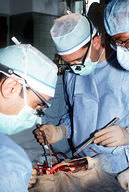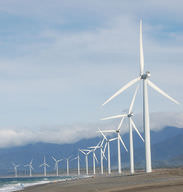# 1.5: Basic and Applied Science

•• Contributed by CK12
• CK12

### Why should we study the rainforest?

Some scientists study problems that seem to have very little impact on our lives. For example, scientists are working to describe every type of plant and animal in the rainforest. What is the purpose? Many of our medicines come from plants and animals of the rainforest. So what medicines have not yet been discovered? There might be new cures to diseases yet to be identified. This is an example of how science can be applied to our lives.

## Basic and Applied Science

Science can be "basic" or "applied." The goal of basic science is to understand how things work—whether it is a single cell, an organism made of trillions of cells, or a whole ecosystem. Scientists working on basic science questions are simply looking to increase human knowledge of nature and the world around us. The knowledge obtained through the study of the subspecialties of the life sciences is mostly basic science.

Basic science is the source of most scientific theories. For example, a scientist that tries to figure out how the body makes cholesterol, or what causes a particular disease, is performing basic science. This is also known as basic research. Additional examples of basic research would be investigating how glucose is turned into cellular energy or determining how elevated blood glucose levels can be harmful. The study of the cell (cell biology), the study of inheritance (genetics), the study of molecules (molecular biology), the study of microorganisms and viruses (microbiology and virology), the study of tissues and organs (physiology) are all types of basic research, and have all generated lots of information that is applied to humans and human health.

Applied science is using scientific discoveries, such as those from basic research, to solve practical problems. For example, medicine, and all that is known about how to treat patients, is applied science based on basic research (Figure below). A doctor administering a drug to lower a person's cholesterol is an example of applied science. Applied science also creates new technologies based on basic science. For example, designing windmills to capture wind energy is applied science (Figure below). This technology relies, however, on basic science. Studies of wind patterns and bird migration routes help determine the best placement for the windmills.Figure $$\PageIndex{1}$$: Surgeons operating on a person, an example of applied science.Figure $$\PageIndex{2}$$: Windmills capturing energy, an example of applied science.

## Summary

• Basic science, such as understanding how cells work, is research aimed at understanding fundamental problems.
• Applied science, such as the medical field, is the application of basic scientific knowledge to solve practical problems.
• Applied science uses and applies information obtained through basic science.

## Explore More

Use the resources below to answer the questions that follow.

### Explore More I

1. What is basic research? Give two examples of basic research.
2. What is applied research? Give two examples of applied research.
3. What is the relationship between basic research and applied science?
4. Why do some scientists believe more emphasis needs to be placed on applied science?

### Explore More II

1. How could basic biomedical research lead to better physicians?
2. What is BMPER? Did its discovery come from basic or applied research? Explain your reasoning fully.

Review

1. What is the difference between basic and applied science?
2. What is an example of applied science?